﻿ 晃荡载荷作用下LNG船B型独立液货舱支撑结构受力分析
 舰船科学技术2019, Vol. 41Issue (8): 17-22PDF

1. 华中科技大学 船舶与海洋工程学院，湖北 武汉 430074;
2. 高新船舶与深海开发装备协同创新中心，上海 200240;
3. 船舶和海洋水动力湖北省重点实验室，湖北 武汉 430074

Force analysis of support structure of LNG independent Type B tank under sloshing loads
DONG Wen1, YUAN Yi1, ZHANG Zheng-yi1,2,3, XIE De1,2,3
1. School of Naval Architecture and Ocean Engineering, Huazhong University of Science and Technology, Wuhan 430074, China;
2. Collaborative Innovation Center for Advanced Ship and Deep-Sea Exploration (CISSE), Shanghai 200240, China;
3. Hubei Key Laboratory of Naval Architecture and Ocean Engineering Hydrodynamics (HUST), Wuhan 430074, China
Abstract: To conduct the force analysis of the support structure of LNG independent Type B tank under sloshing loads, a two-dimensional finite element model with simplified support structure was established. The Abaqus was used to simulate the sloshing and calculate the force of the support structure under different cases based on arbitrary Lagrangian-Eulerian method and bulk modulus reduction technique. The following conclusions are drawn: 1. the periodic rolling motion results in the periodic support structure force; 2. the larger the rolling amplitude, the greater the range of the force variation; 3. in the same density and volume, the range of force variation caused by liquid cargo is greater than solid cargo; 4. the baffles in the cargo tank can reduce the range of force variation. The numerical methods and conclusions in this paper can contribute to the strength and fatigue analysis of the support structure during the preliminary design phase.
Key words: ALE method     bulk modulus reduction     independent type B tank     sloshing
0 引　言

1 研究对象

B型液货舱按形状可分为棱柱形（SPB型）和球形（MOSS型），如图1所示。本文的研究对象是棱柱形液货舱，主要由耐低温的平面板材制成。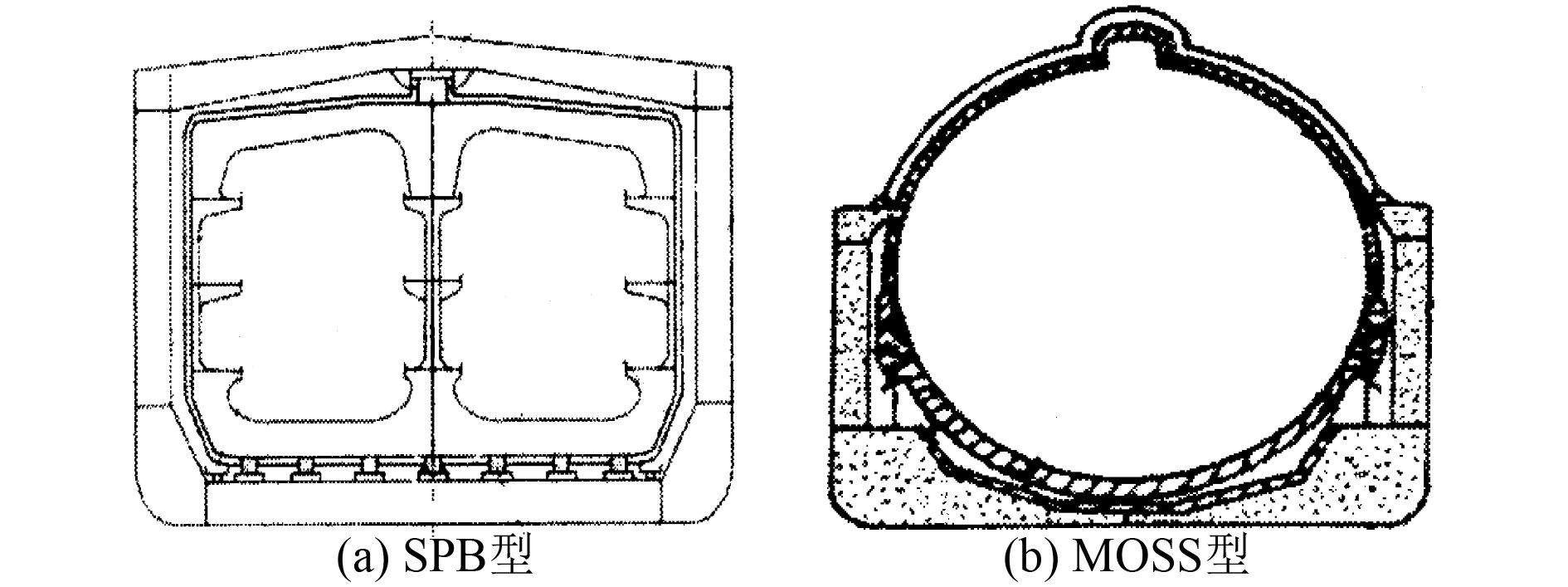图 1 B型独立液货舱 Fig. 1 Independent type B tank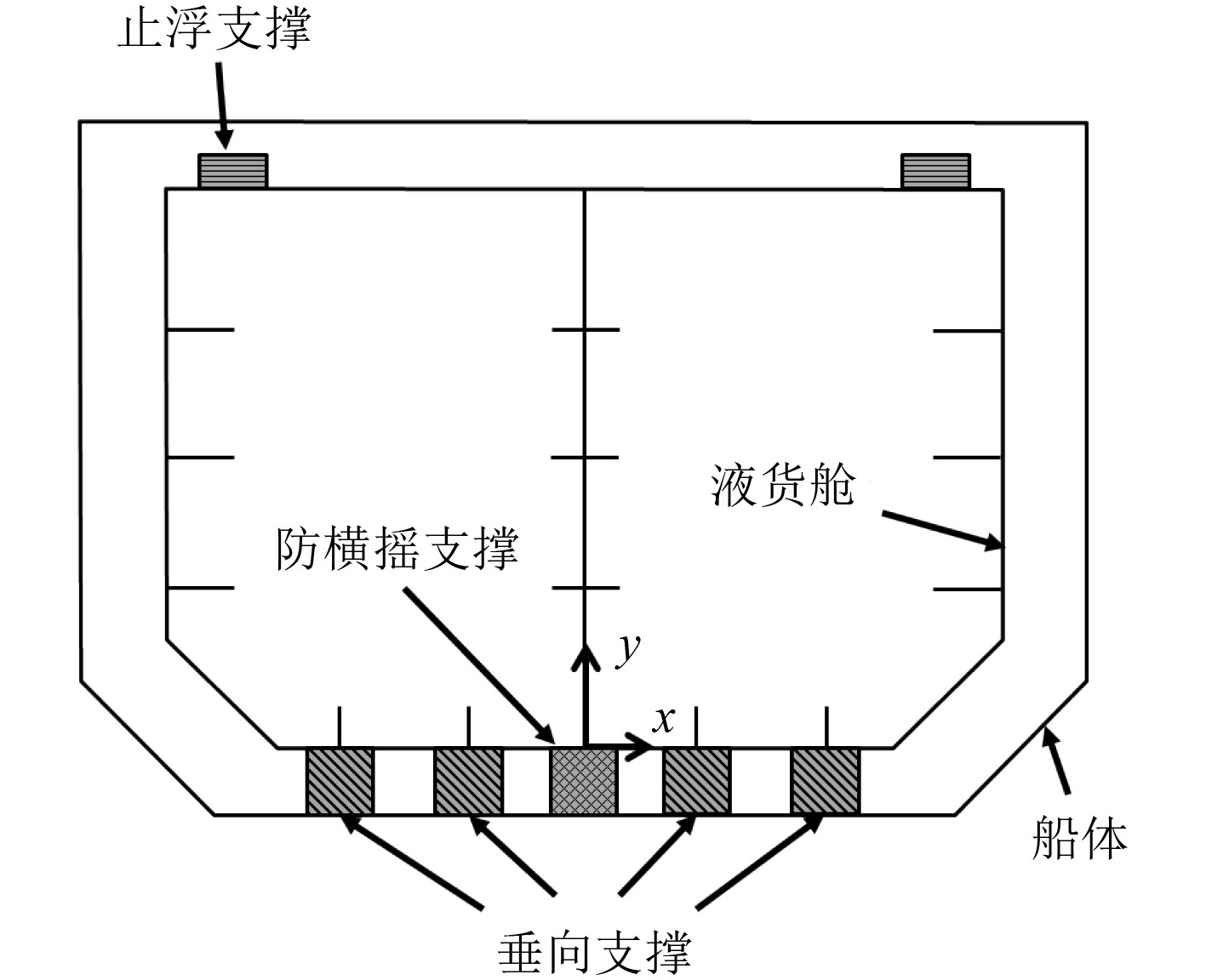图 2 B型独立液货舱支撑结构 Fig. 2 Support structure of independent type B tank
2 数值仿真 2.1 数值方法

 $\frac{{\partial f\left( {{X_i},t} \right)}}{{\partial t}} = \frac{{\partial f\left( {{x_i},t} \right)}}{{\partial t}} + \frac{{\partial f\left( {{x_i},t} \right)}}{{\partial {x_i}}}{w_i} {\text{。}}$ (1)

 $\frac{{\partial \rho }}{{\partial t}} = - \rho \frac{{\partial {v_i}}}{{\partial {x_i}}} - {w_i}\frac{{\partial \rho }}{{\partial {x_i}}}{\text{，}}$ (2)
 $\rho \frac{{\partial {v_i}}}{{\partial t}} = {\sigma _{ij,j}} + \rho {b_i} - \rho {w_i}\frac{{\partial {v_i}}}{{\partial {x_j}}} {\text{，}}$ (3)
 $\rho \frac{{\partial E}}{{\partial t}} = {\sigma _{ij,j}}{v_{i,j}} + \rho {b_i}{v_i} - \rho {w_j}\frac{{\partial E}}{{\partial {x_j}}}{\text{。}}$ (4)

 $p - {p_{ref}} = \mathit{\Gamma} \rho \left( {e - {e_{ref}}} \right){\text{。}}$ (5)

 $\Gamma = \frac{1}{\rho }{\left. {\frac{{\partial p}}{{\partial e}}} \right|_\rho }{\text{。}}$ (6)

Abaqus引入了线性 ${U_S} - {U_P}$ Hugoniot 关系，可将 ${p_{ref}}$ 改写为[11-13]

 ${p_{ref}} = \frac{{{\rho _{ref}}c_0^2\eta }}{{{{\left( {1 - s\eta } \right)}^2}}}{\text{。}}$ (7)

 ${U_S} = {c_0} + s{U_P}{\text{。}}$ (8)

 $K = {\rho _{ref}}c_0^2{\text{，}}$ (9)

 $\Delta t \approx \frac{{{L_{\min }}}}{{{c_d}}}{\text{。}}$ (10)

 ${c_d} = \sqrt {\frac{{E\left( {1 - \mu } \right)}}{{\rho \left( {1 + \mu } \right)\left( {1 - 2\mu } \right)}}}{\text{。}}$ (11)

 $K = \frac{E}{{3\left( {1 - 2\mu } \right)}}{\text{，}}$ (12)

 ${c_d} = \sqrt {K\frac{{3\left( {1 - \mu } \right)}}{{\rho \left( {1 + \mu } \right)}}}{\text{。}}$ (13)

2.2 方法验证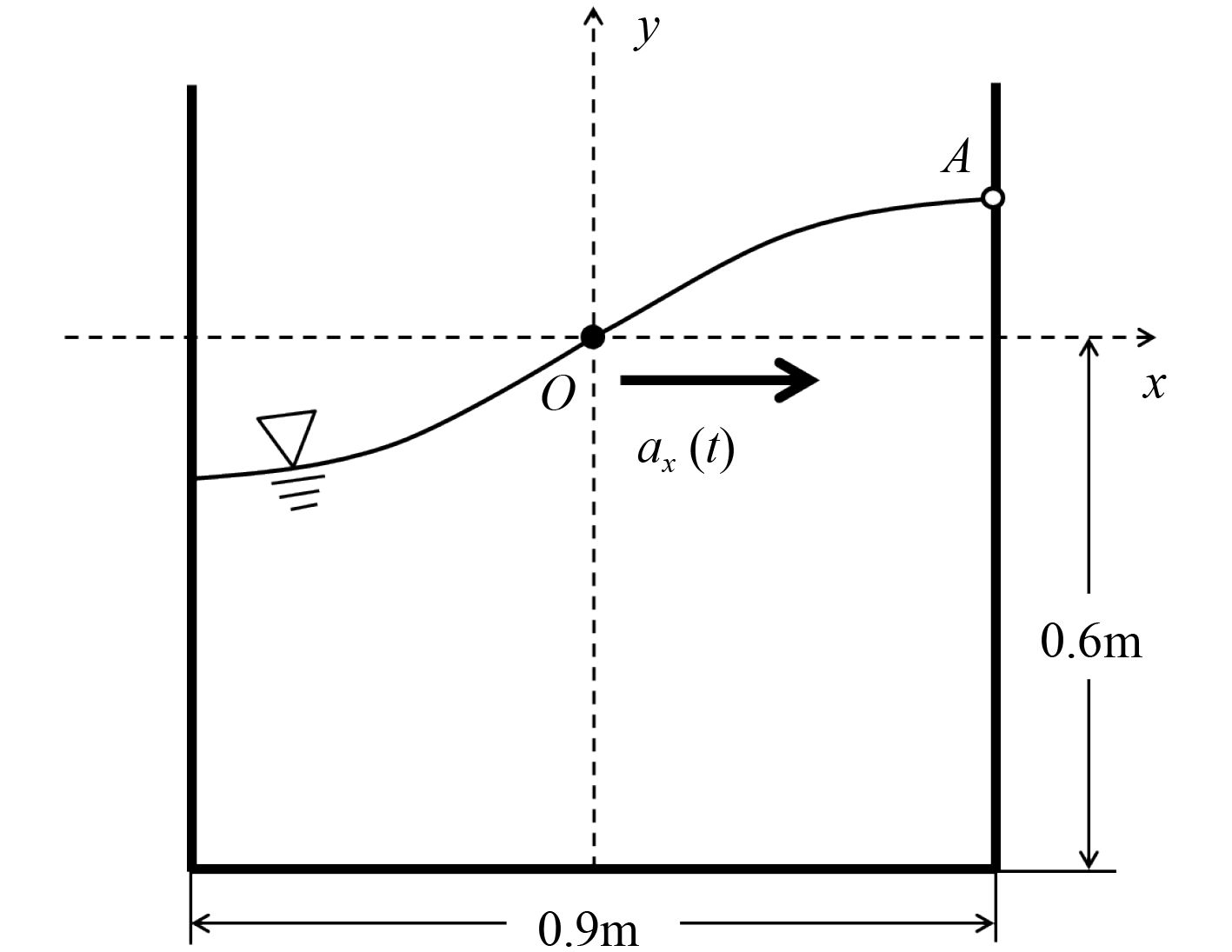图 3 二维矩形容器晃荡算例 Fig. 3 Sloshing case of a two-dimensional container

 ${a_x}\left( t \right) = - {X_0}{\omega ^2}{\rm sin} \omega t{\text{。}}$ (14)表 1 不同模型的计算时间 Tab.1 Computation time of different models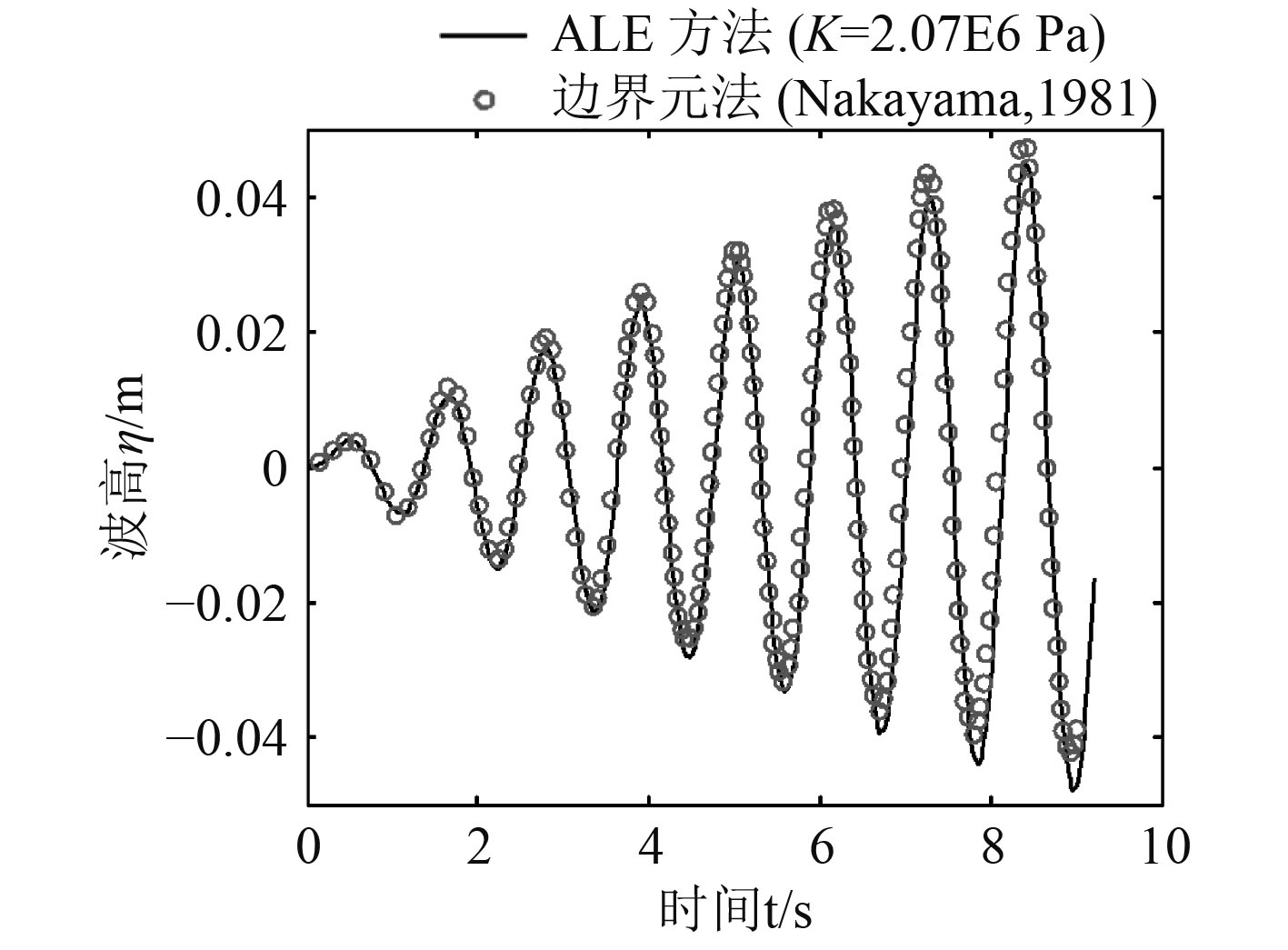图 4 自由液面上A点的波高变化 Fig. 4 Variation of wave height at point A

2.3 有限元模型表 2 支撑结构刚度系数 Tab.2 Stiffness coefficient of support structure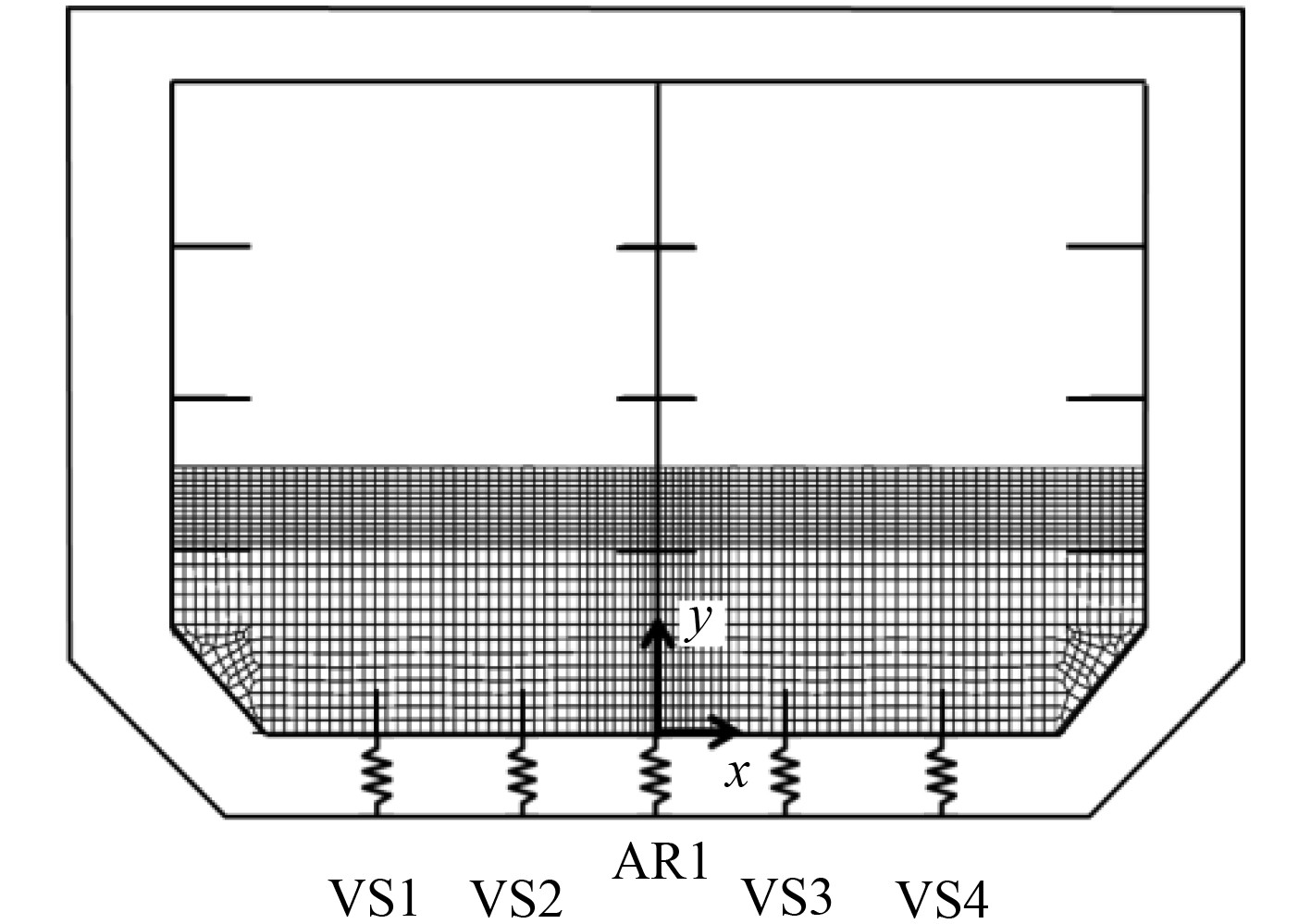图 5 B型独立液货舱有限元模型 Fig. 5 FE model of the independent type B tank表 3 LNG物理属性 Tab.3 Material properties of LNG
2.4 计算工况表 4 计算工况 Tab.4 Computation case

 $\varphi = - {\varphi _0}\sin \omega t{\text{。}}$ (15)

3 计算结果表 5 LC1工况下垂向支撑的作用力 Tab.5 Force on the vertical support structure in LC1

4个垂向支撑的总支持力与液货舱重力的误差小于1%，说明连接单元能够较好的模拟支撑结构，传递作用力。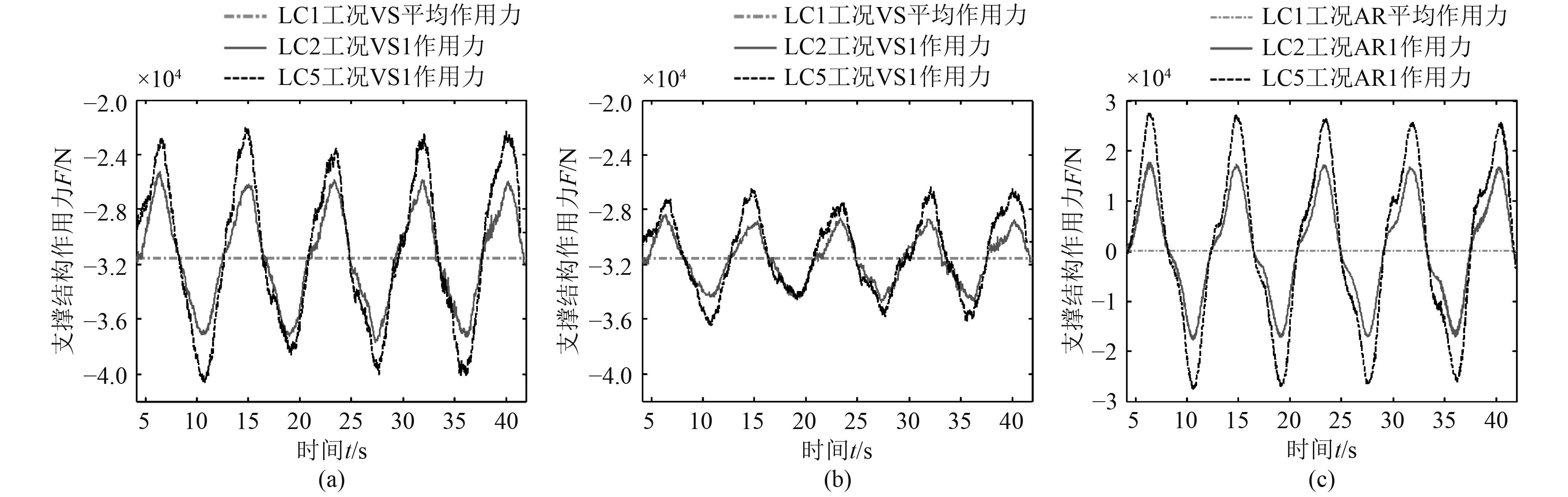图 6 LC2和LC5工况支撑结构作用力对比 Fig. 6 Comparison of force on supports in LC2 and LC5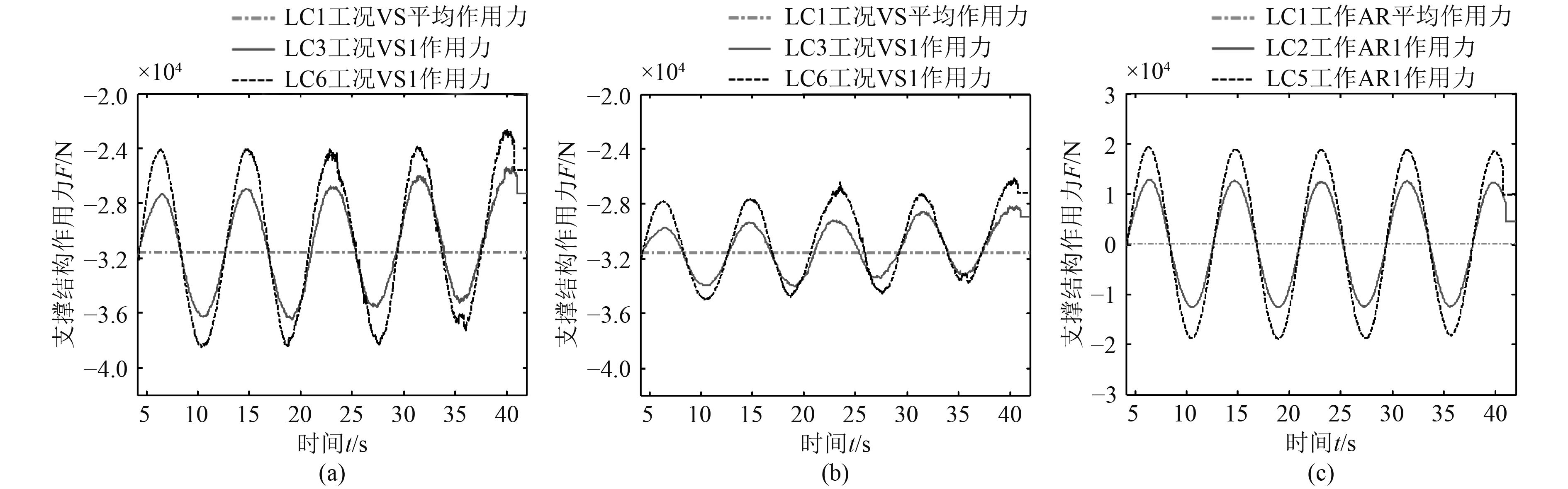图 7 LC3和LC6工况支撑结构作用力对比 Fig. 7 Comparison of forces on supports in LC3 and LC6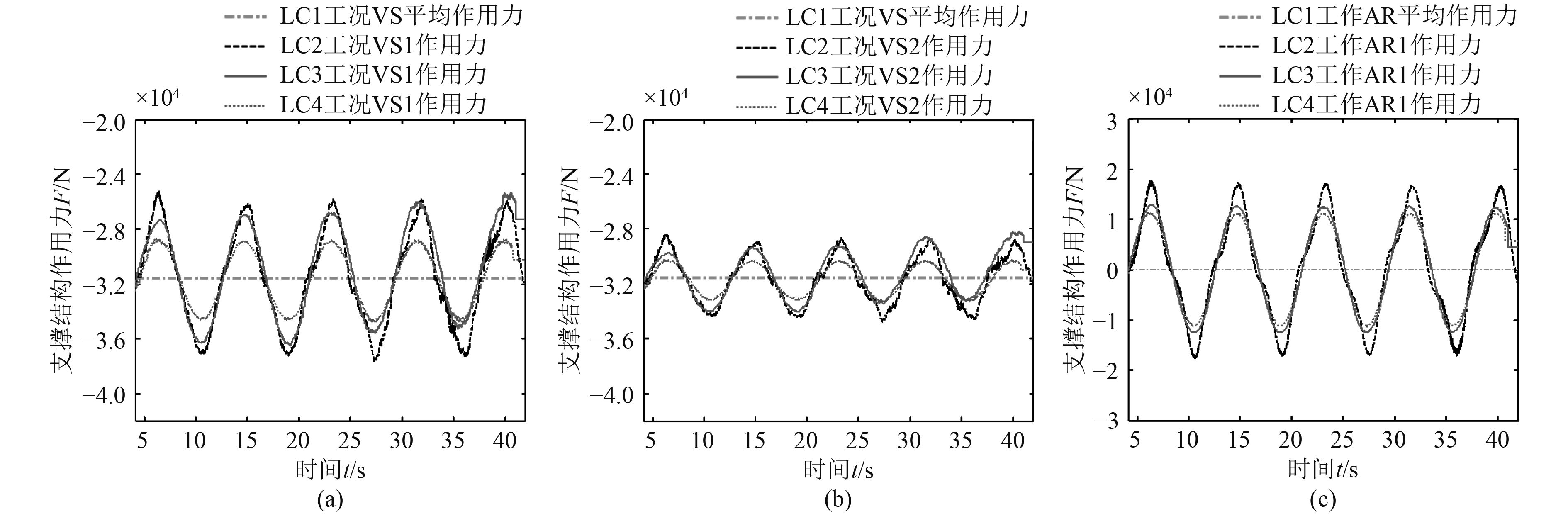图 8 LC2，LC3和LC4工况支撑结构作用力对比 Fig. 8 Comparison of forces on supports in LC2，LC3 and LC4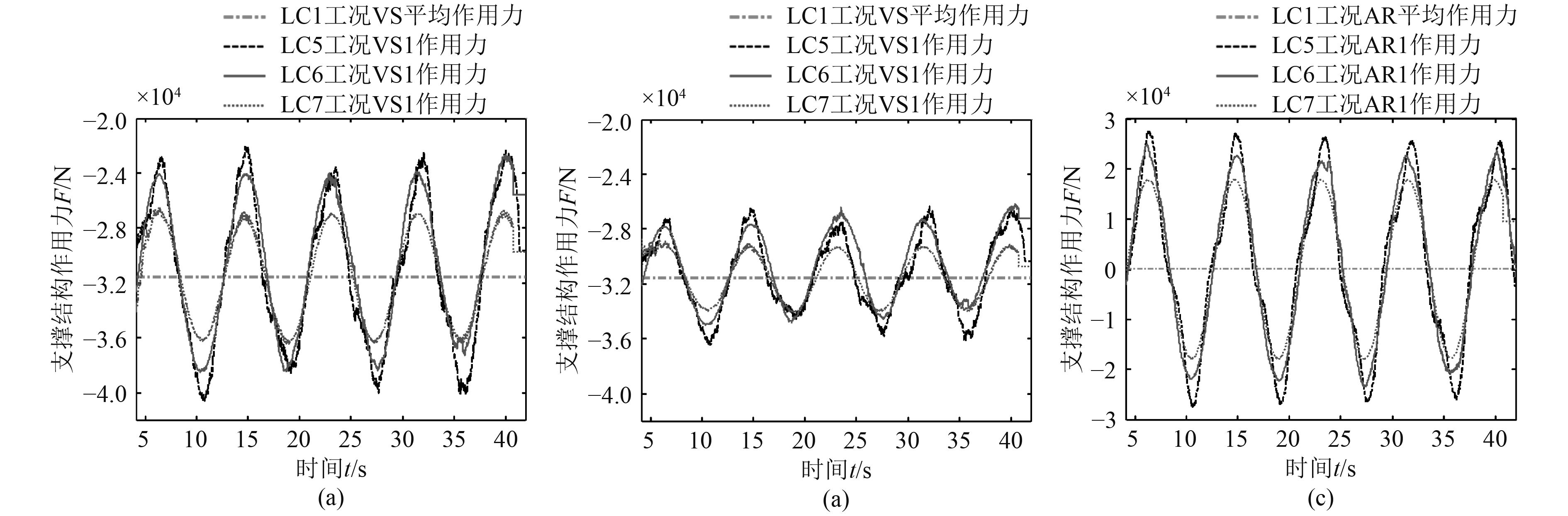图 9 LC5，LC6和LC7工况支撑结构作用力对比 Fig. 9 Comparison of forces on supports in LC5，LC6 and LC7
4 结　语

1）船体做周期性横摇运动时，支撑结构作用力也呈现出周期性变化，且在平衡位置附近波动；

2）船体横摇幅值越大，支撑结构作用力的变化范围越大；

3）由于液舱晃荡，装载液体货物时支撑结构作用力的变化范围大于装载相同密度和体积的固体货物时的变化范围；

4）液货舱内设置挡板能够降低支撑结构作用力的变化范围。

  刘文夫, 薛鸿祥, 唐文勇, 等. 独立B型LNG棱形液舱晃荡载荷数值分析[J]. 船舶工程, 2015(7): 22-25. LIU Wen-fu, XUE Hong-xiang, TANG Wen-yong, et al. Sloshing loads analysis of LNG carrier with independent Type B prismatic tanks[J]. Ship Engineering, 2015(7): 22-25.  IMO, International code for the construction and equipment of ships carrying liquefied gases in bulk[S]. International Maritime Organization (IMO), 2012.  陆志妹, 范佘明. 船舶液舱晃荡研究进展[J]. 船舶与海洋工程, 2010(2): 14–16.  NAKAYAMA T, WASHIZU K. Nonlinear analysis of liquid motion in a container subject to forced pitching oscillation[J]. International Journal for Numerical Methods in Engineering, 1980, 15(8): 1207-1220. DOI:10.1002/nme.1620150808  HIRT CW, NICHOLS BD. Volume of fluid (VOF) method for the dynamics of free boundaries[J]. Journal of Computational Physics, 1981, 39(1): 201-225. DOI:10.1016/0021-9991(81)90145-5  HIRT CW, AMSDEN AA, COOK JL. An arbitrary Lagrangian-Eulerian computing method for all flow speeds[J]. Journal of Computational Physics, 1974, 14(3): 227-253. DOI:10.1016/0021-9991(74)90051-5  王永伟, 王晶. SPB储罐系统设计及特点[J]. 船舶物资与市场, 2007(6): 18–20.  丁峻宏, 金允龙, 王惠. 基于ALE的矿粉货物液化晃荡问题并行数值模拟[J]. 船舶力学, 2015(8): 927-933. DING Jun-hong, JIN Yun-long, WANG Hui. ALE-based parallel numerical simulation for sloshing problem of liquefied ore fines cargo[J]. Journal of Ship Mechanics, 2015(8): 927-933. DOI:10.3969/j.issn.1007-7294.2015.08.006  郑建国. 一般状态方程多流体界面数值方法研究[D]. 合肥: 中国科学技术大学, 2005.  吴宗铎, 严谨, 蒋颉, 等. 基于Mie-Grüneisen状态方程的水下爆炸数值模拟[J]. 舰船科学技术, 2017, 39(13): 29-33. WU Zong-duo, YAN Jin, JIANG Jie, et al. The numerical simulation of underwater explosion based on Mie-Grüneisen equation of state[J]. Ship Science and Technology, 2017, 39(13): 29-33.  ABAQUS 6.10. Analysis user’s manual[M]. Dassault Systèmes, 2010.  莫建军, 孙承纬. 200 GPa压力范围内铝和铜的等熵压缩线计算[J]. 高压物理学报, 2006, 20(4): 386-390. MO Jian-jun, SUN Cheng-wei. Compression isentropes of aluminum and copper up to 200 GPa[J]. Chinese Journal of High Pressure Physics, 2006, 20(4): 386-390. DOI:10.3969/j.issn.1000-5773.2006.04.008  冉宪文. 混合物高压物态方程的理论研究[D]. 长沙: 国防科学技术大学, 2002.  程利冬, 王忠金. 高速锤击体积变形过程的有限元分析[J]. 哈尔滨工程大学学报, 2008, 29(2): 183-188. CHENG Li-dong, WANG Zhong-jin. Finite element analysis of the deformation process in high impact hammering[J]. Journal of Harbin Engineering University, 2008, 29(2): 183-188. DOI:10.3969/j.issn.1006-7043.2008.02.016  NAKAYAMA T, WASHIZU K. The boundary element method applied to the analysis of two-dimensional nonlinear sloshing problems[J]. International Journal for Numerical Methods in Engineering, 1981, 17(11): 1631-1646. DOI:10.1002/nme.1620171105  杜显赫. 预应力LNG储罐在地震作用下的流固耦合数值模拟[D]. 沈阳: 沈阳工业大学, 2011.